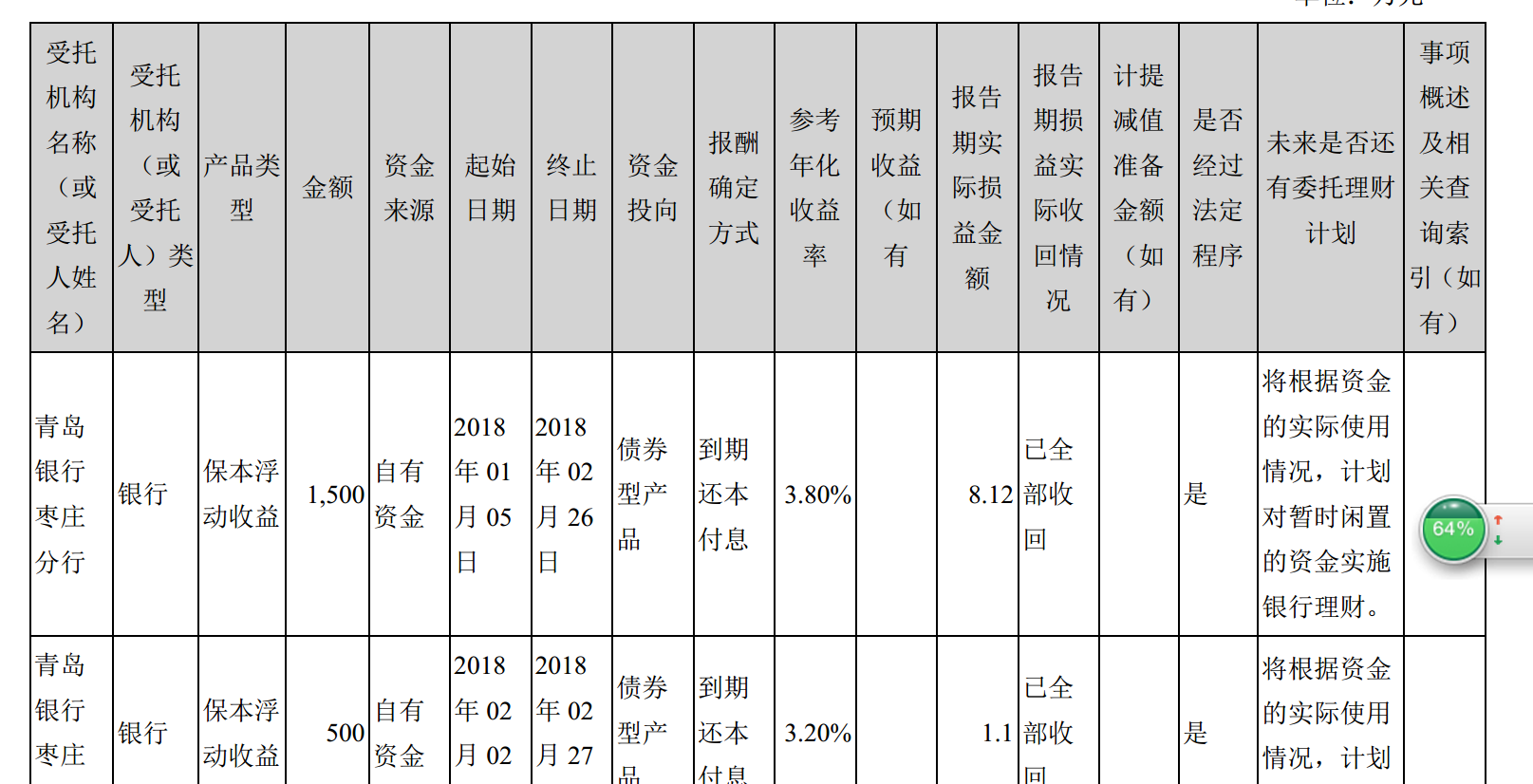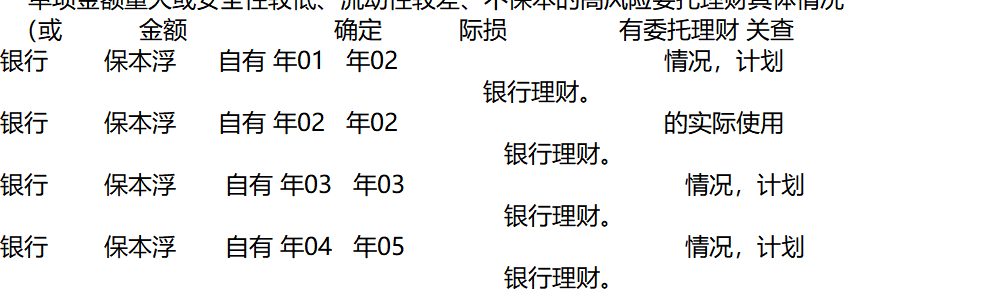2019-07-31 16:21

10

# 希望达到：用代码扣下如下图示的（含有关键词：XX）的表格。``````问题：由于格式转换导致表格格式信息丢失，一句话会被分割在好几行，因此无法扣下完整的句子。（如下图所示）
````````````import re
import os

filePath = 'D:/XML/reporttxt'
namelist=os.listdir(filePath)   #获取文件名
j=0
while(j<len(namelist)):          #遍历多个文件（pdf）

f = open('D:/XML/reporttxt/'+namelist[j],encoding='utf-8')
ff= open('D:/XML/reporttxtlast/'+namelist[j],"w+")
j=j+1
i=0
lines=f.readlines()     #遍历每一行
while(i<len(lines)):
line=(str)(lines[i])
res=re.search(r'保本',line)    #匹配关键词
res2=re.search(r'理财',line)
i=i+1
if(res!=None):      #如果匹配到了关键词，则把整行写入新txt文件
#print(line)
ff.write(line)
elif(res2!=None):
ff.write(line)
f.close()
ff.close()
``````

``````问题：写入txt的内容不会漏信息，但会失去表格的格式，再加上每个格子里本身就会带有很多换行符，导致可读性很差
``````
``````import re
import os
from docx import Document

filePath = 'D:/XML/reportword'
namelist=os.listdir(filePath)
doc=Document('D:/XML/reportword/'+namelist)   #读取的word文件
fff= open('D:/XML/reporttxtlast/'+namelist.rstrip(".docx")+'yoyo.txt',"w+")     #扣下来的信息放入这个txt文件

for k in range(0,len(doc.tables),1):
table=doc.tables[k]
good=False    #false代表没有在这个表格里发现关键词，true则有
chart=[[0 for col in range(len(table.rows.cells))] for row in range(len(table.rows))]            #初始化chart用来放置表格里的文字
for m in range(0,len(table.rows),1):     #每一行
row=table.rows[m]
for n in range(0,len(row.cells),1):     #行中的每一格
cell=row.cells[n]
chart[m][n]=str(cell.text)

chart[m][n]=chart[m][n].strip("\n").strip('\n')   #去除换行符（问题！？？这一步还是会遗留一些\n除不干净，不懂为啥）

if (re.search(r'受托机构',cell.text)!=None):    #匹配到关键词
good=True

if(good):     关键词匹配成功，把当下的表格写入txt（问题！？？不知道怎么写入txt能保留原本表格的样式）

for m in chart:
for n in m:
print(n)
fff.write(n)
fff.close()
``````

• 点赞
• 写回答
• 关注问题
• 收藏
• 复制链接分享
• 邀请回答

• 点赞 评论 复制链接分享